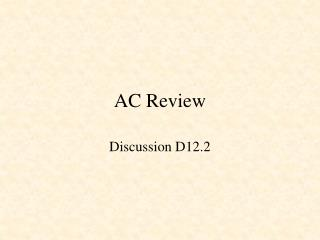DownloadDownload PresentationAC Review

AC Review

Télécharger la présentationAC Review

- - - - - - - - - - - - - - - - - - - - - - - - - - - E N D - - - - - - - - - - - - - - - - - - - - - - - - - - -
Presentation Transcript

1. AC Review Discussion D12.2

2. Passive Circuit Elements i i i + -

3. Energy stored in the capacitor The instantaneous power delivered to the capacitor is The energy stored in the capacitor is thus

4. Energy stored in the capacitor Assuming the capacitor was uncharged at t = -, and knowing that represents the energy stored in the electric field established between the two plates of the capacitor. This energy can be retrieved. And, in fact, the word capacitor is derived from this element’s ability (or capacity) to store energy.

5. Parallel Capacitors Thus, the equivalent capacitance of N capacitors in parallel is the sum of the individual capacitances. Capacitors in parallel act like resistors in series.

6. Series Capacitors The equivalent capacitance of N series connected capacitors is the reciprocal of the sum of the reciprocals of the individual capacitors. Capacitors in series act like resistors in parallel.

7. Energy stored in an inductor The instantaneous power delivered to an inductor is The energy stored in the magnetic field is thus

8. Series Inductors The equivalent inductance of series connected inductors is the sum of the individual inductances. Thus, inductances in series combine in the same way as resistors in series.

9. Parallel Inductors The equivalent inductance of parallel connected inductors is the reciprocal of the sum of the reciprocals of the individual inductances.

10. Complex Numbers Complex Plane imag A real j Euler's equation: j measured positive counter-clockwise Note:

11. Relationship between sin and cos

12. Comparing Sinusoids Note: positive angles are counter-clockwise

13. A phasor is a complex number that represents the amplitude and phase of a sinusoid. Phasors XM j Recall that when we substituted in the differential equations, the cancelled out. We are therefore left with just the phasors

14. - VR + + VL - Impedance Impedance Units = ohms Note that impedance is a complex number containing a real, or resistive component, and an imaginary, or reactive, component.

15. - VR + + VL - Admittance Admittance Units = siemens susceptance conductance

16. Im V V I I Re I in phase with V Im V I V Re I lags V I Im V I V I Re I leads V

17. We see that if we replace Z by R the impedances add like resistances. Impedances in series add like resistors in series Impedances in parallel add like resistors in parallel

18. Voltage Division But Therefore

19. Instantaneous Power Note twice the frequency

20. Average Power Purely reactive circuit Purely resistive circuit

21. Effective or RMS Values We define the effective or rms value of a periodic current (voltage) source to be the dc current (voltage) that delivers the same average power to a resistor. root-mean-square

22. Effective or RMS Values and Using

23. Ideal Transformer - Voltage The input AC voltage, v1, produces a flux f This changing flux through coil 2 induces a voltage, v2 across coil 2

24. Ideal Transformer - Current Magnetomotive force, mmf f The total mmf applied to core is For ideal transformer, the reluctance R is zero.

25. Ideal Transformer - Impedance Load impedance Input impedance Turns ratio

26. Ideal Transformer - Power Power delivered to primary Power delivered to load Power delivered to an ideal transformer by the source is transferred to the load.

27. Force on current in a magnetic field Force on moving charge q -- Lorentz force Current density, j, is the amount of charge passing per unit area per unit time. N = number of charges, q, per unit volume moving with mean velocity, v. j Force per unit length on a wire is

28. B Force out commutator i + - i brushes Force in X Rotating Machine

29. Back emf X X B B Force out r i a q l + - b i Force in

30. B Force out commutator i + - i brushes Force in X Back emf

31. X X X X Armature with four coil loops S N

32. Motor Circuit Power and Torque